Next: Electromagnetic Waves in Unmagnetized Up: Dispersive Waves Previous: Dispersive Waves

# Pulse Propagation

Consider a one-dimensional wave pulse,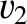(771)

made up of a linear superposition of cosine waves, with a range of different wavenumbers, all traveling in the positive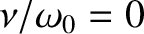-direction. The angular frequency,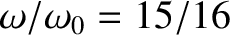, of each of these waves is related to its wavenumber,, via the so-called dispersion relation, which can be written schematically as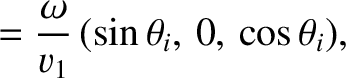(772)

In general, this relation is derivable from the wave disturbance's equation of motion. Up to now, we have only considered sinusoidal waves that have linear dispersion relations of the form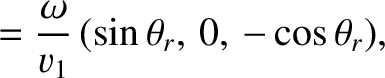(773)

whereis a constant. The above expression immediately implies that such waves have the same phase velocity,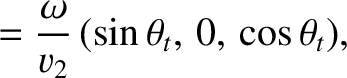(774)

irrespective of their frequencies. Substituting Equation (773) into Equation (771), we obtain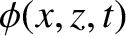(775)

which is the equation of a wave pulse that propagates in the positive-direction, at the fixed speed, without changing shape. (See Chapter 9.) The above analysis seems to suggest that arbitrarily shaped wave pulses generally propagate at the same speed as sinusoidal waves, and do so without dispersing or, otherwise, changing shape. In fact, these statements are only true of pulses made up of superpositions of sinusoidal waves with linear dispersion relations. There are, however, many types of sinusoidal wave whose dispersion relations are nonlinear. For instance, the dispersion relation of sinusoidal electromagnetic waves propagating through an unmagnetized plasma is (see Section 10.2)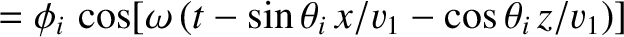(776)

where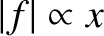is the speed of light in vacuum, and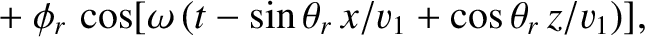is a constant, known as the plasma frequency, that depends on the properties of the plasma. [See Equation (798).] Moreover, the dispersion relation of sinusoidal surface waves in deep water is (see Section 10.7)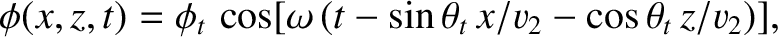(777)

where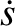is the acceleration due to gravity,the surface tension of water, and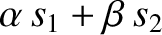the mass density. Sinusoidal waves that satisfy nonlinear dispersion relations, such as (776) or (777), are known as dispersive waves, as opposed to waves that satisfy linear dispersion relations, such as (773), which are called non-dispersive waves. As we saw previously, a wave pulse made up of a linear superposition of non-dispersive sinusoidal waves, all traveling in the same direction, propagates at the common phase velocity of these waves, without changing shape. How does a wave pulse made up of a linear superposition of dispersive sinusoidal waves evolve in time?

Suppose that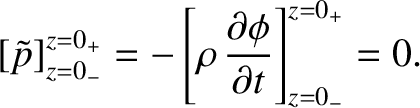(778)

In other words, the function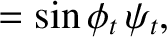in Equation (771) is a Gaussian, of characteristic width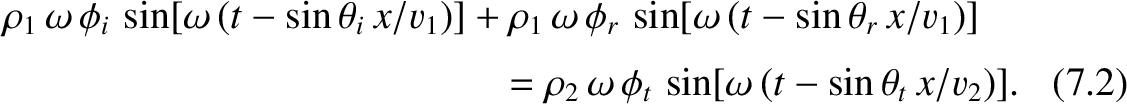, centered on wavenumber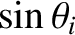. It follows, from the properties of the Gaussian function, thatis negligible for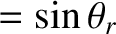. Thus, the only significant contributions to the wave integral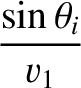(779)

come from a small region of-space centered on. Let us Taylor expand the dispersion relation,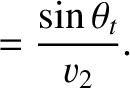, about. Neglecting second-order terms in the expansion, we obtain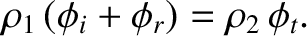(780)

It follows that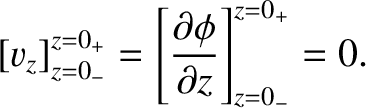(781)

where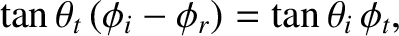, and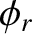(782)

is a constant with the dimensions of velocity. Ifis sufficiently small then the neglect of second-order terms in the expansion (781) is a good approximation, and Equation (779) becomes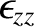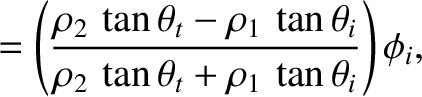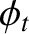(783)

where use has been made of a standard trigonometric identity. (See Appendix B.) The integral involving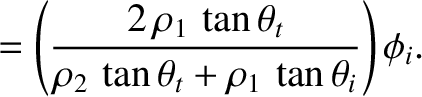is zero, by symmetry. Moreover, an examination of Equations (712)-(715) reveals that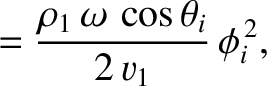(784)

where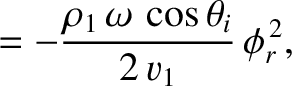. Hence, by analogy with this expression, (783) reduces to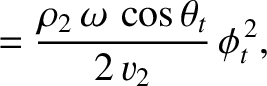(785)

This is the equation of a wave pulse, of wavenumber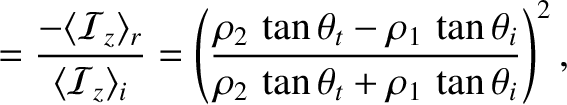, and angular frequency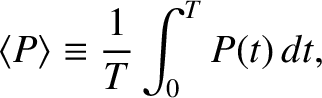, with a Gaussian envelope, of characteristic width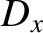, whose peak (which is located by setting the argument of the exponential to zero) has the equation of motion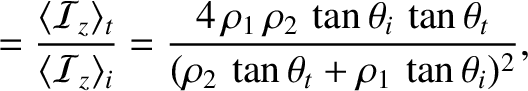(786)

In other words, the pulse peak--and, hence, the pulse itself--propagates at the velocity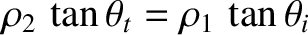, which is known as the group velocity. In the case of non-dispersive waves, the group velocity is the same as the phase velocity (because, if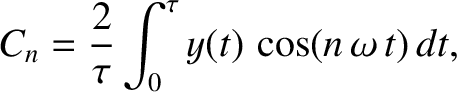then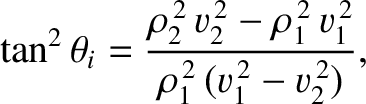). However, for the case of dispersive waves, the two velocities are, in general, different.

Equation (785) indicates that, as the wave pulse propagates, its envelope remains the same shape. Actually, this result is misleading, and is only obtained because of the neglect of second-order terms in the expansion (781). If we keep more terms in this expansion then we can show that the wave pulse does actually change shape as it propagates. However, this demonstration is most readily effected by means of the following simple argument. The pulse extends in Fourier space from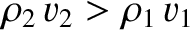to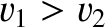, where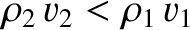. Thus, part of the pulse propagates at the velocity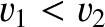, and part at the velocity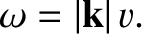. Consequently, the pulse spreads out as it propagates, because some parts of it move faster than others. Roughly speaking, the spatial extent of the pulse in real space grows as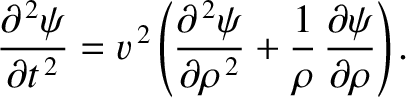(787)

where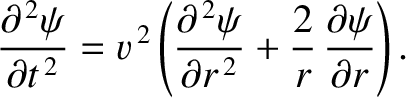is the extent of the pulse at. Hence, from Equation (782),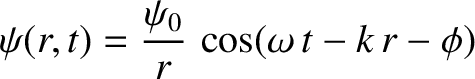(788)

We, thus, conclude that the spatial extent of the pulse grows linearly in time, at a rate proportional to the second derivative of the dispersion relation with respect to(evaluated at the pulse's central wavenumber). This effect is known as pulse dispersion. Incidentally, there is no pulse dispersion in a non-dispersive medium, because, by definition,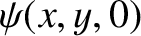in such a medium. In summary, a wave pulse made up of a linear superposition of dispersive sinusoidal waves, with a range of different wavenumbers, propagates at the group velocity, and also gradually disperses as time progresses.Next: Electromagnetic Waves in Unmagnetized Up: Dispersive Waves Previous: Dispersive Waves
Richard Fitzpatrick 2013-04-08